# Gaussian Processes and Hedge for infinite armed bandits

Warning: this post is under construction.

## 1 Introduction

• Today we will cover a “non-convex” setting.
• The setting is that of adversarial BO.
• We will get results for infinite arm Bandits. Crucially we will introduce known adversarial actions.

## 2 Bayesian Optimization

Bayesian Optimization (BO) is a variant of the Multi-Armed Bandit setting from the previous post. In particular, it considers:

• Infinite arms: That is, the decision set is now some subset of the euclidean space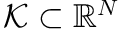.
• Stochastic arms: The function is not changing between iterations but instead observed through some noisy observation,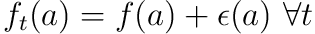. The noise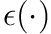is usually assumed to be homogenously Gaussian, i.e.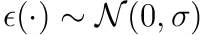.

It tightens the assumption in MAB slightly by also assuming that the function is expensive to evaluate. This motivates use of methods for the point selection which has high computational budget such as Gaussian Processes.

A popular example of BO is hyperparameter optimization, e.g.:

•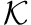is the hyperparameters of a neural network such as depth, width, activation function etc.
•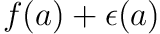is the measured test accuracy of the neural network after training with the added noisy due to the randomness of the initialization. Notice that the evaluation is indeed very expensive.
##### Regret

A small complication notation-wise is that BO usually considers maximization of a function instead of minimization. We are thus usually interested in the cumulative regret defined aswhere our algorithm selects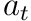.

This setting is well-studied but we will now complicate the setup by considering an adverserial setting instead of stochastic arms.

## 3 GP-UCB

(GP-UCB) Select the next point as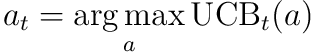where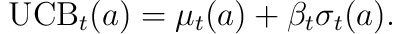## 4 GP-MW

##### Setting

In the context of OCO we are thus considering an infinite armed limited information setting with adversarially picked functions. Note that we have mentioned no assumption of convexity of the functions.In the work of  they crucially assume known adversarial actions. This is intuitively reasonable, since we need to exploit correlation between our actions in the infinite arm setting. Without known adversarial actions this correlation would be unknown.

This assumption allows us to formulate a rather simple algorithm: 1) At each time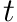construct the acquisition function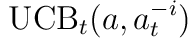using all previous observations. 2) Notice that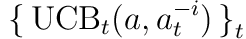is known on the full space in contrast to the loss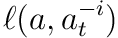. Thus the Hedge algorithm from the previous post can be applied directly. This is made precise below.

(GP-MW)To understand exactly why this works, let us dig into the analysis.

## 5 Regret Analysis

The main ingredient will be characterizing the function by the posterior GP.

(Confidence lemma) Let

• unknown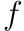have bounded RKHS norm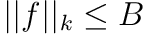.
• the noise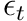be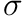-sub-Gaussian in our observation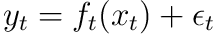.
•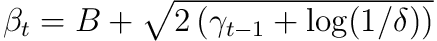.

Then with at least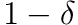the following holds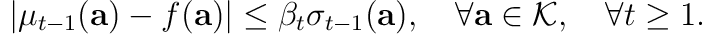where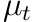and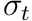is the posterior mean and variance of our GP model conditioned on all observations up till time.

In other words we can say that the true function lies within some confidence bound computed by our Gaussian Process model with high probability.

### 5.1 Split into two terms

Our main problem in the analysis is treating the limited information setting. Using the Confidence Lemma we will see how we can instead treat a full information setting.

##### Using The Confidence Lemma

With this we can upper and lower bound the loss with high probabilityThis section is almost entirely lifted from the original paper.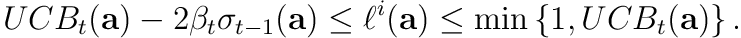This particular way of writing the bounds allows us to split the regret into two parts,The first term (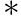) can now be treated as a full information setting where the function is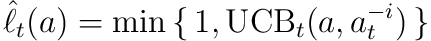while the second part (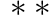) can be analysed independent of the algorithmThis trick is in fact more general and has also been applied to Reinforcement Learning .

.

#### 5.1.2 Bounding with Hedge

We cannot apply our tools from OCO directly tosince it is non-convex in general. However, we can linearize the problem by optimizing a probability distribution instead.

Given an Online Optimization problem with decisions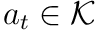and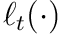loss we can lift it into a linear problem in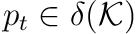,where expectation is taken w.r.t. the randomness of the algorithm.

Applying this to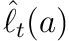allows us to bound () in the regret using Hedge from the previous post even thoughis non-convexNotice that even though we recast it in terms of a mixed strategy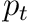the loss we incur is still w.r.t. to a single arm selection. This can seem slightly confusing. However, this slight modification of Hedge has no effect on the regret, sinceis still constructed based on full information. Thus, the expectation of the loss over the randomness of the algorithm is equivalent to the linear loss on the mixed strategy,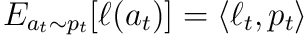.

.

Using the high probability bound relying on Azume-Hoeffding inequility from the previous post we get with probabilitythat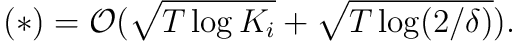#### 5.1.3 Bounding the Variance Term

What remains is to bound (). This part is what requires us to have known adversarial actions. Otherwise we can intuitively not ensure that the variance will decrease.

#### 5.1.4 Final Regret Bound

(GP-MW finite arms regret bounds) Let

•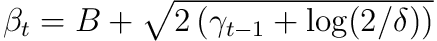•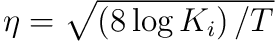then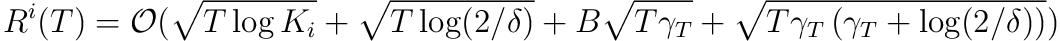### 5.2 Discretization

So far the algorithm has been defined in terms of a finite number of arms (even if the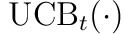on which the mixed strategy is computed is in fact continuous). This does not help solve the non-convex infinite arm setting which we set out for originally. One trivial solution to this taken in  is to discretize the space.

For this we just have to be careful to cover our space densely enough so that the regret will not suffer. The Lipschitz assumption onwill ensure this, e.i. if points are close to the discretization their loss will also be close.

To make this precise:

(GP-MW infinite arms regret bounds) Discretize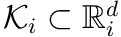with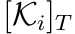where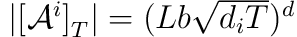so that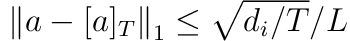.

### 5.3 Final Remarks

In the OCO framing we have thus treated non-convex function in the bandit setting when the decision set is a simplex (finite or infinite).

For more general non-convex problems for online optimization we face slightly different problems. First, how do we even define regret? After all we can do arbitrarily bad since in the non-convex setting we are only promised to converge to a local minimum. Two recent papers deals with these questions .

I have intentionally left out some details of the canonical BO/GP part to keep distractions to a minimum — but with the obvious danger of being imprecise. For precisely specified assumptions of each lemma and statement please see .

 P. G. Sessa, I. Bogunovic, M. Kamgarpour, and A. Krause, “No-regret learning in unknown games with correlated payoffs,” in Advances in neural information processing systems 32, H. Wallach, H. Larochelle, A. Beygelzimer, F. dAlché-Buc, E. Fox, and R. Garnett, Eds. Curran Associates, Inc., 2019, pp. 13602–13611 [Online]. Available: http://papers.nips.cc/paper/9514-no-regret-learning-in-unknown-games-with-correlated-payoffs.pdf

 C.-A. Cheng, R. T. des Combes, B. Boots, and G. Gordon, “A reduction from reinforcement learning to no-regret online learning.” 2019.